Sun, P., Liang, K., and Wang, S. (2023). “Comparative analysis of mechanical properties for wood frame and reinforced concrete frame based on deformation energy decomposition method,” BioResources 18(3), 7124-7142.

#### Abstract

As a typical orthotropic material, the mechanical properties of wood in the parallel and perpendicular-to-grain directions are very different. Based on mathematical orthogonality, mechanical force balance condition, and energy comparability, the deformation energy decomposition method of planar wood element is proposed, and then the quantitative and visual analysis of the basic deformation performance of wood structure is realized. The basic deformation performance of wood structure and isotropic structure is analyzed using the deformation energy decomposition method, and the seismic performance of wood frame structure and concrete frame structure is compared. The results show that the lateral resistance and beam ductility of wood frame are greater than those of concrete frame under seismic load. However, the reduction of deformation energy proportion of wood frame beam leads to greater deformation energy of the frame column, so it is suggested to take targeted strengthening measures to the bottom of the wood frame column and the joint area.

Comparative Analysis of Mechanical Properties for Wood Frame and Reinforced Concrete Frame Based on Deformation Energy Decomposition Method

Panxu Sun,a Kaixuan Liang,a,* and Shuxia Wang b

As a typical orthotropic material, the mechanical properties of wood in the parallel and perpendicular-to-grain directions are very different. Based on mathematical orthogonality, mechanical force balance condition, and energy comparability, the deformation energy decomposition method of planar wood element is proposed, and then the quantitative and visual analysis of the basic deformation performance of wood structure is realized. The basic deformation performance of wood structure and isotropic structure is analyzed using the deformation energy decomposition method, and the seismic performance of wood frame structure and concrete frame structure is compared. The results show that the lateral resistance and beam ductility of wood frame are greater than those of concrete frame under seismic load. However, the reduction of deformation energy proportion of wood frame beam leads to greater deformation energy of the frame column, so it is suggested to take targeted strengthening measures to the bottom of the wood frame column and the joint area.

DOI: 10.15376/biores.18.4.7124-7142

Keywords: Wood; Orthotropic anisotropy; Frame structure; Deformation energy decomposition; Seismic performance

Contact information: a: School of Civil Engineering, Zhengzhou University, Zhengzhou, 450001, China; b: School of Materials Science and Engineering, Zhengzhou University of Aeronautics, Zhengzhou 450046, China; *Corresponding author: 202011211030241@gs.zzu.edu.cn

INTRODUCTION

As a natural biological material, wood has the advantages of environmental protection, renewable nature, excellent material properties, and abundant availability (Brischke 2021; Chen et al. 2021; Beims et al. 2022). Therefore, wood is widely used in building structures and other fields (Zhou et al. 2014; Bagheri and Doudak 2020). Wood is a typical natural polymer anisotropic material. Because of the arrangement of internal cells and tissues, wood has significantly different physical and mechanical properties in different directions (Liu et al. 2020; Dong et al. 2022). The ratio of elastic modulus in parallel-to-grain direction to perpendicular-to-grain direction of wood can reach 30 times. This material property makes a great difference in the deformation performance between the wood structure and the traditional concrete structure. If the isotropic theory is used to analyze and calculate the wood structure, it is difficult to describe the real mechanical behavior of the structure. Therefore, it is necessary to fully consider the influence of the modulus of the material in different directions in the wood structure.

Wood structure has a long history of development. However, as a modern wood structure, its related research is relatively less than that of reinforced concrete structure. In recent years, most of the research topics on wood at home and abroad are at the material or component level. At present, the research on the material properties of wood mainly have focused on materials such as dimension lumber, laminated veneer lumber (LVL), and glued laminated wood. The research methods include experimental research, numerical simulation, and theoretical analysis (Tumenjargal et al. 2020; Xie et al. 2021; Moya et al. 2023). Research on wood component has also been more systematic, and the research work has covered wood beams, columns, shear walls, plates, etc. (Aicher et al. 2016; Lahr et al. 2017; Zhou et al. 2021; Acuña et al. 2023; Xu et al. 2023). Due to the complexity of the anisotropic material constitutive model, it brings many difficulties to the numerical simulation of the wood structure. Therefore, a sufficient number of experiments are needed as the basis for the research work, and probability statistical analysis method is needed to analyze its various performance indicators (Zhang et al. 2020; Islam et al. 2022; Ventura et al. 2023). At present, research on the deformation performance of the complete wood structure is more dependent on the experiment and field test, and the corresponding theoretical analysis and numerical simulation are relatively weak. Therefore, a more simple and effective method is needed to analyze the basic deformation properties of wood structures.

Weak parts of structure may be damaged first in an earthquake. Several examples (Seyedkhoei et al. 2019; Göçer 2020; Nale et al. 2021) show that in an earthquake, the damage to a structure is mostly caused by a domino effect. Excessive deformation energy is concentrated in key areas, resulting in local damage, and ultimately leading to the overall damage or collapse of the structure. The design and reinforcement of the structure should match the basic macroscopic mechanical responses, such as tensile, bending, and shear, etc. (Chen et al. 2023; Li and Deng 2023; Pejatović et al. 2023). The deformation decomposition method is a structural analysis method that can decompose the comprehensive deformation into basic macroscopic deformation (Shi and Goodman 1989; Jin et al. 2011; Zhang and Hoa 2014; Wang et al. 2022). However, the existing deformation decomposition method is only applicable to isotropic materials and cannot be used to analyze orthotropic wood structures. Therefore, this paper proposes an improved deformation energy decomposition method based on mathematical orthogonality, mechanical force balance, and physical parameters of orthotropic materials. Compared with the traditional finite element simulation, which can only analyze the microscopic deformation such as strain and shear strain, the deformation energy decomposition method can further quantify the macroscopic deformation information such as tension and compression, shear and bending, and provide the corresponding theoretical basis for the design and reinforcement of wood structures.

The content of this paper is mainly divided into four parts. The second part introduces the deformation energy decomposition method of wood structure in detail. In the third part, the basic deformation performance of wood structure and isotropic structure is analyzed by deformation energy decomposition method, and the seismic performance of wood frame and concrete frame is compared. The fourth part provides some conclusions and the prospect of future research work.

DEFORMATION ENERGY DECOMPOSITION METHOD OF WOOD STRUCTURE

The finite element model of the wood structure is established and the node displacement vector of each element is extracted. The basic deformation energy of the element can be calculated by using the node displacement vector and material properties, and the deformation energy decomposition diagram and deformation energy cloud diagram can be drawn according to the size of the deformation energy. The finite element analysis part is completed by ANSYS software, and the subsequent energy calculation work is realized by MATLAB software. The whole deformation decomposition work is completed by writing an interface program for the two software programs. The specific workflow of ANSYS and MATLAB is shown in Fig. 1.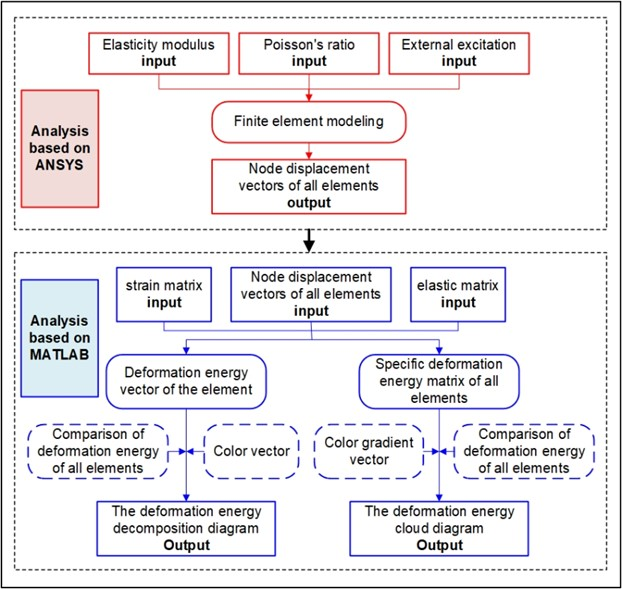Fig. 1. Algorithm of deformation energy decomposition subroutine

Wood has different physical and chemical properties in the parallel and perpendicular-to-grain directions. Compared with the isotropic materials, such as steel and concrete, the main difference of the elastic theory of wood lies in the constitutive equation. For planar structures, isotropic materials have three independent elastic parameters. The shear modulus is G. The anisotropic material has four independent elastic parameters, namely, the X-axial elastic modulus EX, the X-axial elastic modulus EY, the main Poisson’s ratio μXY, and the shear modulus GXY.

The comprehensive deformation of a planar 4-node square wood element can be decomposed into 8 kinds of mutually orthogonal basic deformation and rigid body displacement, including X-axial tensile and compressive deformation, Y-axial tensile and compressive deformation, X-axial bending deformation, Y-axial bending deformation, shear deformation, X-axial rigid body displacement, Y-axial rigid body displacement, and rigid body rotation.

Square elements are used to divide the planar wood structure, and the node displacement of element j can be calculated and extracted by finite element analysis. The displacements of the four nodes are sorted according to the node order in Fig. 2a, and then the node displacement vector uj corresponding to Fig. 2b is given in Eq. 1,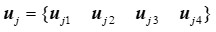(1)

where uji is the displacement vector of the i-th node for the j-th element, namely: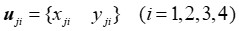(2)

where xji and yji are the displacement values along the X axis and Y axis, respectively.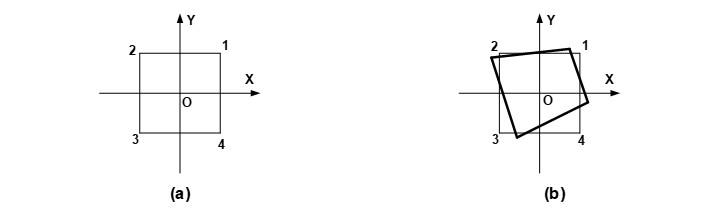Fig. 2. Planar square wood element (a) Schematic diagram; (b) Comprehensive deformation

According to anisotropic elasticity theory (Skrzypek and Ganczarski 2015), the constitutive relationship of wood is given as Eq. 3,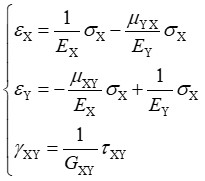(3)

where EX and EY are the elastic modulus in X and Y axis directions, respectively; μXY is the main Poisson’s ratio; GXY is the shear modulus; εX and σX are the normal strain and stress in the X axis direction, respectively; εY and σY are the normal strain and stress in the Y axis direction, respectively; γXY and τXY are the shear strain and stresses.

The relationship between Poisson’s ratio and elastic modulus is given as Eq. 4: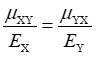(4)

The shear elastic modulus (Zienkiewicz and Taylor 2000) can be expressed as: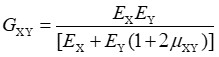(5)

The stiffness matrix is given as Eq. 6,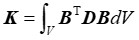(6)

where B is the strain matrix; D is the elastic modulus matrix.

The comprehensive node load vector Fj can be calculated by Eq. 7: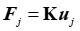(7)

Based on balance conditions of mechanical force and mathematical orthogonality, five basic deformation and three basic rigid body displacement load conditions for square wood element are constructed (Fig. 3).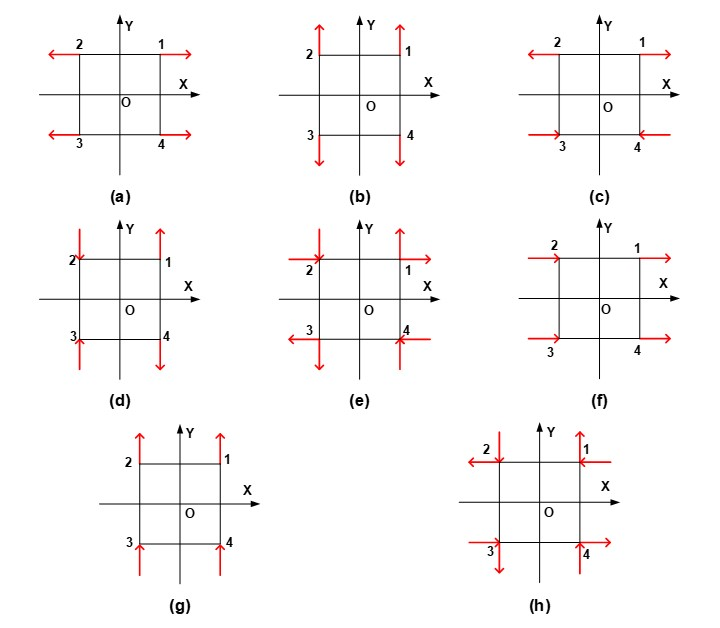Fig. 3. Basic deformation and rigid body displacement load conditions of square wood element: (a) X-axial tensile and compressive deformation; (b) Y-axial tensile and compressive deformation; (c) X-axial bending deformation; (d) Y-axial bending deformation; (e) Shear deformation; (f) X-axial rigid body displacement; (g) Y-axial rigid body displacement; (h) Rigid body rotation

The node load mode vectors f1 to f8 corresponding to each load condition can be obtained according to Fig. 3, and the load mode matrix composed of them is given as Eq. 8,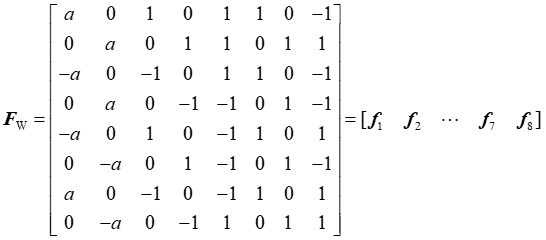(8)

where f1 is the node load mode vector of X-axial tension and compression deformation of the element, f2 is the node load mode vector of Y-axial tension and compression deformation, f3 is the node load mode vector of Y-axial tension and compression deformation, f4 is the node load mode vector of Y-axial bending deformation, f5 is the node load mode vector of shear deformation, f6 is the node load mode vector of X-axial rigid body displacement, f7 is the node load mode vector of Y-axial rigid body displacement, and f8 is the node load mode vector of rigid body rotation. When a = 1, f1 and f2 are the node load mode vector of X- and Y-axial tensile deformation, respectively. When a = −1, f1 and f2 are the node load mode vector of X- and Y-axial compression deformation, respectively.

The load mode matrix F satisfies mathematical orthogonality, that is Eq. 9: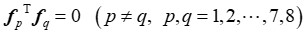(9)

The load mode projection coefficient vector ξ of element j can be obtained by Eq. 10: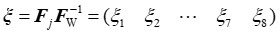(10)

Under the load conditions in Fig. 3, the corresponding 5 basic deformations and 3 rigid body displacements can be obtained, as shown in Fig. 4. According to Figure 4, the node displacement mode vectors d1 to d8 corresponding to load mode vectors can be constructed. The node displacement mode matrix is composed as Eq. 11 below: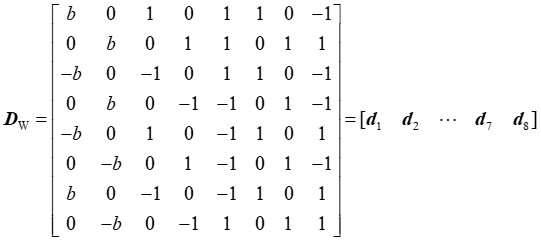(11)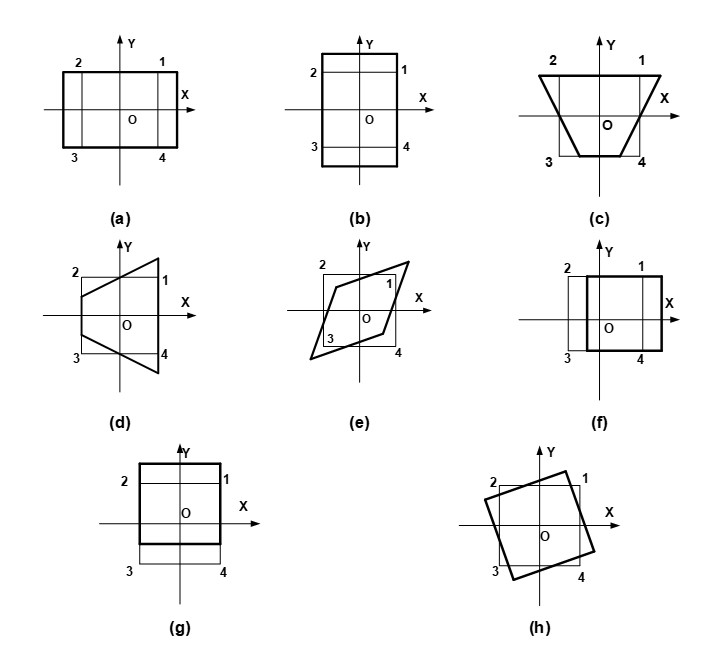Fig. 4. Basic deformation and rigid body displacement of planar square wood element: (a) X-axial tensile and compressive deformation; (b) Y-axial tensile and compressive deformation; (c) X-axial bending deformation; (d) Y-axial bending deformation; (e) Shear deformation; (f) X-axial rigid body displacement; (g) Y-axial rigid body displacement; (h) Rigid body rotation

When b = 1, d1 and d2 are the node displacement mode vector of X- and Y-axial tensile deformation, respectively. When b = −1, d1 and d2 are the node displacement mode vector of X- and Y-axial compression deformation.

The node displacement mode matrix F satisfies mathematical orthogonality, that is Eq. 12 below: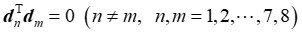(12)

The node displacement mode projection coefficient vector η of element j can be obtained by the following Eq. 13: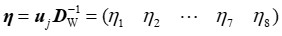(13)

If we ignore the influence of rigid body displacement, then five basic deformation energies of element j can be calculated by Eq. 14,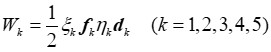(14)

where W1 is the X-axial tensile and compressive deformation energy, W2 is the Y-axial tensile and compressive deformation energy, W3 is the X-axial bending deformation energy, W4 is the Y-axial bending deformation energy, and W5 is the shear deformation energy.

The values of each basic deformation energy are compared, and the main basic deformation energy of the element can be obtained. The energy is scalar, and it is necessary to judge the tensile and compressive state of the element according to the positive and negative of the node displacement mode projection coefficient η1 and η2. For example, a positive η1 indicates that the element is in the X-axial tensile state, while a negative sign indicates that the element is in the X-axial compression state. For the convenience of analysis, each basic deformation energy corresponds to a color and abbreviation, as shown in Table 1. Using this method to decompose the deformation energy of all elements, the deformation energy decomposition diagram of the structure can be drawn.

To analyze the distribution of the specific basic deformation energy in the wood structure, the values of the specific basic deformation energy of all elements are divided into intervals. The deformation energy cloud diagram of the structure can be obtained by corresponding the elements in each interval to a color grade.

Table 1. Colors and Abbreviations Corresponding to Each Basic Deformation Energy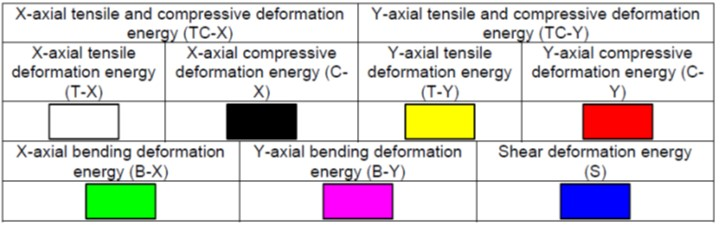SEISMIC PERFORMANCE ANALYSIS OF WOOD FRAME

Deformation Performance Analysis of Wood Frame

The ratio of elastic modulus in parallel-to-grain direction to perpendicular-to-grain direction of wood can reach 30 times, so the parallel-to-grain direction of wood has better resistance to deformation compared to the perpendicular-to-grain direction. Take the three-story wood frame WF1 as an example, the dimensions of which are shown in Fig. 5. The elastic modulus of the parallel-to-grain direction of wood is EL =12600 MPa, the elastic modulus of the perpendicular-to-grain direction is ET = 420 MPa, the principal Poisson’s ratio is μLT = 0.4, and the density is ρ = 440 kg/m3. For frame beams, the elastic modulus in the X-axis direction is EL, and the elastic modulus in the Y-axis direction is ET. For the frame column, the elastic modulus in the X-axis direction is ET, and the elastic modulus in the Y-axis direction is EL. The seismic fortification intensity is selected as 8°, class II site, and the second group of seismic design group. The load condition of WF1 are calculated according to the equivalent base shear method, as shown in Fig. 5.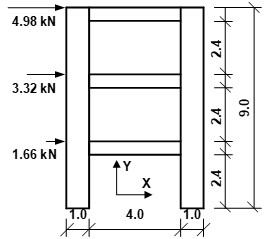Fig. 5. Structural dimensions and force conditions of frames WF1, F1, and F2

The dimensions and load conditions of isotropic frames F1 and F2 are the same as WF1. The elastic modulus of F1 and F2 are EL and ET, respectively. The Poisson’s ratio is 0.4, and the density is 440 kg/m3. The deformation energy decomposition is carried out on the three frame structures respectively, and the corresponding deformation energy decomposition diagram and basic deformation energy cloud diagram are drawn, as shown in Figs. 6 and 7. Among them, the positive and negative energy values in the tensile and compressive deformation energy cloud diagram only represent the tensile and compressive states of the element.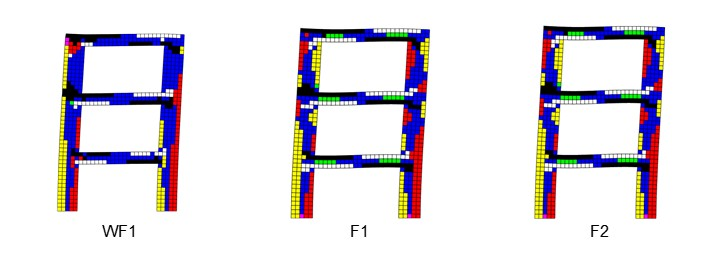Fig. 6. Deformation energy decomposition diagram of frames WF1, F1, and F2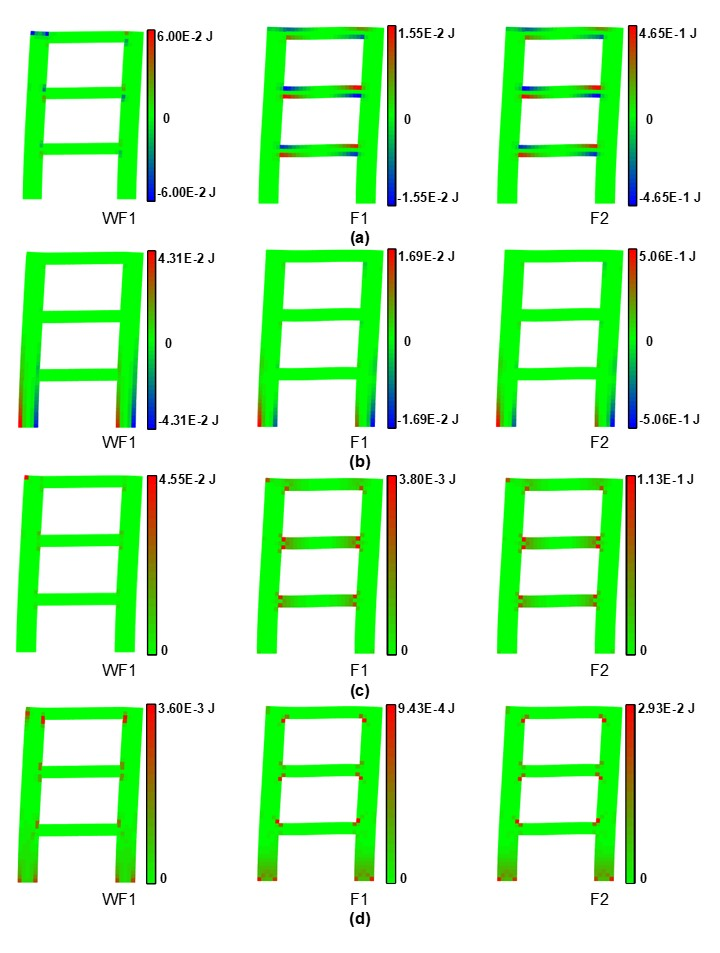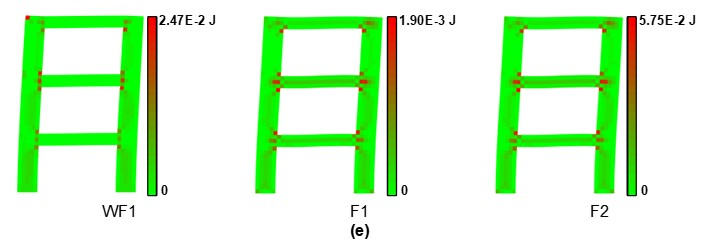Fig. 7. Deformation energy cloud diagram of frames WF1, F1, and F2: (a) X-axial tension and compressive deformation energy; (b) Y-axial tension and compressive deformation energy; (c) X-axial bending deformation energy; (d) Y-axial bending deformation energy; (e) Shear deformation energy

Figure 6 shows that the deformation energy decomposition results of isotropic frames F1 and F2 are the same, because the color of the deformation energy decomposition diagram represents the relative sizes of different deformation energies of the same element. That is, the color in the deformation energy decomposition diagram of two frame structures at the same position is the same, but the size of the basic deformation energy is not necessarily the same. The difference of deformation energy can be reflected by the basic deformation energy cloud diagrams in Fig. 7. Compared with isotropic frame, the area dominated by shear deformation energy (blue) in the deformation energy decomposition diagram of wood frame WF1 is larger.

Figure 7 shows that the distribution of basic deformation energy of F1 and F2 is similar under the same load. The basic deformation energy of each element in F1 is smaller than that in F2, while the basic deformation energy of each element in WF1 is between F1 and F2. This is because the stiffness of F1 is the largest and that of F2 is the smallest among the three frames. Under the same load condition, the deformation of frame F2 is the largest, so its basic deformation energy is also the largest. In addition, the deformation energy of the wood beam under seismic load is small, and the deformation energy is mainly concentrated in the wood column. Compared with F1 and F2, the areas with larger shear deformation energy in WF1 are mainly located in beam-column joints and wood columns. The shear deformation energy in the wood beam is small, and there is almost no area where the X-axial tension and compression deformation energy and the X-axial bending deformation energy are large.

Under linear elastic conditions, the total deformation energy of the structure is the sum of the deformation energy of all elements. The deformation energy of the beams and columns of the three frames are analyzed, respectively. The deformation energy of the beam (or column) can be obtained by summing the deformation energy of the corresponding element, and the corresponding deformation energy proportion can be obtained by dividing the deformation energy of the beam (or column) by the total deformation energy of the structure. The results are shown in Figs. 8 and 9, respectively.

From Fig. 8, it can be seen that the basic deformation energy of the beam of WF1 is smaller than that of F1 and F2, and the proportion of the deformation energy of the beam of WF1 is 40.84% lower than that of F1 and F2. Compared with isotropic beams, the proportion of shear deformation energy of wood beams decreases 1.13%, and the proportion of X-axial bending deformation energy is almost 0.

As shown in Fig. 9, compared with isotropic frames F1 and F2, the deformation energy proportion of columns of wood frame F1 increases 40.84%. Among them, the X-axial tensile and compressive deformation energy and X-axial bending deformation energy proportion of wood frame column are greater than that of isotropic frame F1 and F2. This is because the elastic modulus of the perpendicular-to-grain direction (X-axial) of the wood column is much smaller than that of the parallel-to-grain direction (Y-axial), resulting in relatively large lateral deformation of the wood frame.

In addition, the proportion of shear deformation energy of wood columns increases 16.1%, which is consistent with the result of shear deformation energy cloud diagram in Fig. 7. Therefore, compared with isotropic frames, the ductility of wood frame columns is reduced.

In summary, the orthogonal anisotropy of wood should be fully considered in the analysis of wood structure to ensure the rationality and accuracy of the calculation.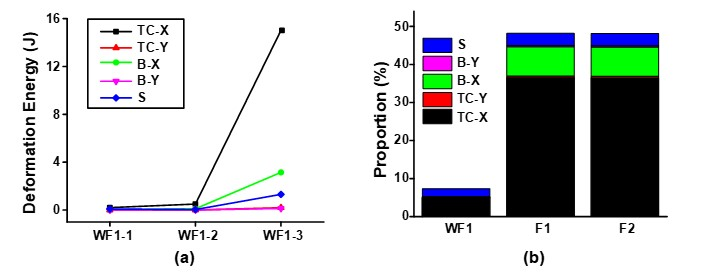Fig. 8. Deformation performance of beams: (a) Deformation energy; (b) The proportion of the deformation energy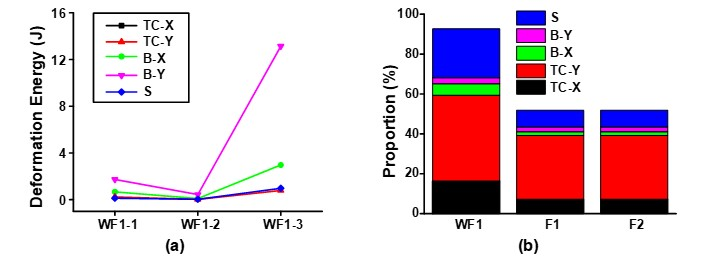Fig. 9. Deformation performance of columns (a) Deformation energy; (b) The proportion of the deformation energy

Comparative Analysis of Wood Frame and Concrete Frame

Wood and concrete are the most commonly used biomaterials and artificial materials in the construction field, respectively. Due to significant differences in material properties, it is necessary to adopt different seismic design methods for wood and concrete structures. Taking the wood frame and concrete frame as an example, the structural requirements of beams and columns are summarized according to the following standards: Code for Design of Concrete Structures, GB/T 50010 (2010), Technical Specification for Concrete Structures of Tall Buildings, JGJ 3 (2010), Standard for Design of Timber Structures, GB/T 50005 (2017), and General Code for Timber Structures, GB/T 55005 (2021), as shown in Table 2.

The section height of the beam is 0.3 m, and the span of the beam is the maximum of the concrete frame structure (18 times the section height). The specific dimension of the structure is shown in Fig. 10. The dimension of the wood frame WF2, and the concrete frame CF both meet the structural requirements. Wood frame WF2 has the same material properties as WF1, as previously mentioned. The elastic modulus of concrete is EC = 30000 MPa, Poisson’s ratio μC = 0.2, and density ρC = 2500 kg/m3. The seismic fortification intensity is selected as 8°, class II site, and the second group of seismic design group. The load conditions of wood frame WF2 and concrete frame CF are calculated according to the equivalent base shear method, as shown in Fig. 10.

Table 2. Structural Requirements of Beams and Columns in Concrete Frame Structure and Wood Structure *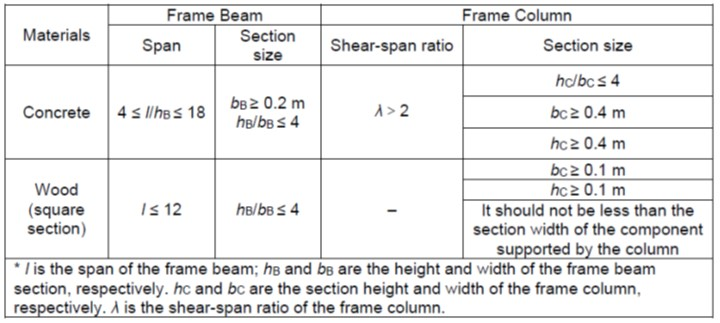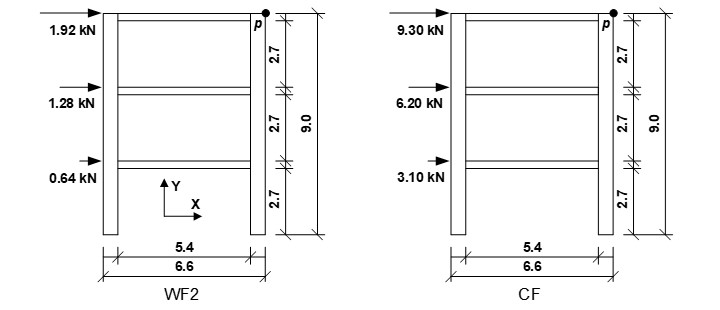Fig 10. Structural dimensions and load conditions of wood frame WF2 and concrete frame CF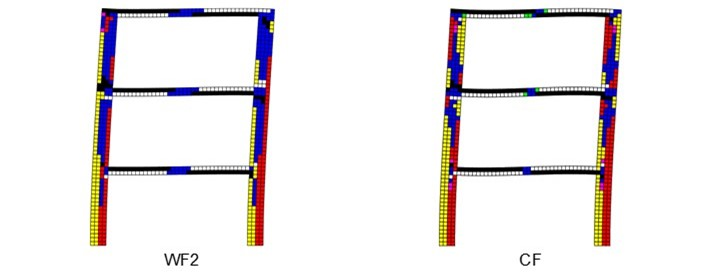Fig. 11. Deformation energy decomposition diagram of frames WF2 and CF

The deformation energy decomposition of wood frame WF2 and concrete frame CF are carried out, and the corresponding deformation energy decomposition diagram and deformation energy cloud diagram are shown in Figs. 11 and 12